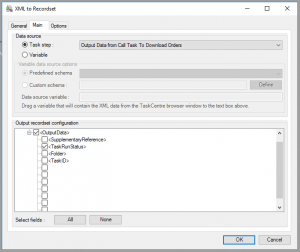The task can run in two modes:

• Synchronous
• QueueQueue
This adds the task to the queue, to be scheduled at a future point in time

Synchronous
This queues the task and awaits a response.

Sometimes in Taskcentre you wish to know if a task you have called has failed. This is useful in cases where there’s a queue of objects which require some work completing and you are processing these in a batch. Examples include:

• Invoices to send
• Sales Orders to process
• etc

The issue with calling tasks Synchronously is that you cannot easily tell if the task being run has failed or not, however by analysing the output of the task, you can record this information and make decisions accordingly.

Here’s the output of calling this task:

```<DataOutput>
<OutputData>
<SupplementaryReference />
<fileName>PO128.doc</fileName>
<filePath>C:\Temp\File_Test</filePath>
</OutputData>
</DataOutput>```

The “TaskRunStatus” flag is the important part here. If the value is “2”, then the task completed successfully. Value “4” is a failure.

Using the “XML To RecordSet” tool, each results from the “Call Task” can be mapped to a Recordset:From there, we can then use the “VBScript” tool to loop around all the RecordSet entries:Here’s the VBScript:

```If ThisStep.RecordSource("OutputData_TaskRunStatus") = 2 Then
Variables("SuccessCount") = Variables("SuccessCount") + 1
Else
Variables("FailCount") = Variables("FailCount") + 1
End If
```

From there, you can use these variables within your other steps. For example, here’s a HTML document which is posted to HipChat using code here.

```Variables("Message") = "<B>Task Completion</B>"
Variables("Message") = Variables("Message") & "<BR/>Success: " & Variables("SuccessCount")
Variables("Message") = Variables("Message") & "<BR/>Fail: " & Variables("FailCount")```